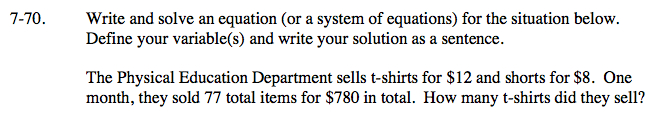Home > CAAC > Chapter 7 > Lesson 7.2.2 > Problem7-70

7-70.

Write and solve an equation (or a system of equations) for the situation below. Define your variable(s) and write your solution as a sentence. Homework Help ✎

The Physical Education Department sells t-shirts for $12 and shorts for$8. One month, they sold 77 total items for \$780 in total. How many t-shirts did they sell?Let t = the number of t-shirts
and s = the number of shorts.

Have one equation explain the relationship between the number of t-shirts and shorts.
Have the other equation explain the relationship between the price of t-shirts and shorts.

Use the elimination method to solve for t.